### PLOT Statement

PLOT <yvariable*xvariable> <=symbol> <…yvariable*xvariable> <=symbol> </ options> ;

The PLOT statement is used with line printer and traditional graphics. See the PLOTS= option for information about using ODS graphics to create modern statistical graphics.

The PLOT statement in PROC REG displays scatter plots with yvariable on the vertical axis and xvariable on the horizontal axis. Line printer plots are generated if the LINEPRINTER option is specified in the PROC REG statement; otherwise, the traditional graphics are created. Points in line printer plots can be marked with symbols, while global graphics statements such as GOPTIONS and SYMBOL are used to enhance the traditional graphics. Note that the plots you request by using the PLOT statement are independent of the ODS graphical displays (see the section ODS Graphics) that are available in PROC REG.

As with most other interactive statements, the PLOT statement implicitly refits the model. For example, if a PLOT statement is preceded by a REWEIGHT statement, the model is recomputed, and the plot reflects the new model.

If there are multiple MODEL statements preceding a PLOT statement, then the PLOT statement refers to the latest MODEL statement.

The PLOT statement cannot be used when a TYPE=CORR, TYPE=COV, or TYPE=SSCP data set is used as input to PROC REG.

You can specify several PLOT statements for each MODEL statement, and you can specify more than one plot in each PLOT statement.

#### Specifying Yvariables, Xvariables, and Symbol

More than one yvariable*xvariable pair can be specified to request multiple plots. The yvariables and xvariables can be as follows:

• any variables specified in the VAR or MODEL statement before the first RUN statement

• keyword., where keyword is a regression diagnostic statistic available in the OUTPUT statement (see Table 79.7). For example,

plot predicted.*residual.;


generates one plot of the predicted values by the residuals for each dependent variable in the MODEL statement. These statistics can also be plotted against any of the variables in the VAR or MODEL statements.

• the keyword OBS. (the observation number), which can be plotted against any of the preceding variables

• the keyword NPP. or NQQ., which can be used with any of the preceding variables to construct normal P-P or Q-Q plots, respectively (see the section Construction of Q-Q and P-P Plots for more information)

• keywords for model fit summary statistics available in the OUTEST= data set with _TYPE_= PARMS (see Table 79.7). A SELECTION= method (other than NONE) must be requested in the MODEL statement for these variables to be plotted. If one member of a yvariable*xvariable pair is from the OUTEST= data set, the other member must also be from the OUTEST= data set.

The OUTPUT statement and the OUTEST= option are not required when their keywords are specified in the PLOT statement.

The yvariable and xvariable specifications can be replaced by a set of variables and statistics enclosed in parentheses. When this occurs, all possible combinations of yvariable and xvariable are generated. For example, the following two statements are equivalent:

plot (y1 y2)*(x1 x2);
plot y1*x1 y1*x2 y2*x1 y2*x2;


The statement

plot;


is equivalent to respecifying the most recent PLOT statement without any options. However, the line printer options COLLECT, HPLOTS=, SYMBOL=, and VPLOTS=, described in the section Line Printer Plots, apply across PLOT statements and remain in effect if they have been previously specified.

Options used for the traditional graphics are described in the following section; see Line Printer Plots for more information.

The display of traditional graphics is described in the following paragraphs, the options are summarized in Table 79.7 and described in the section Dictionary of PLOT Statement Options.

Several line printer statements and options are not supported for the traditional graphics. In particular the PAINT statement is disabled, as are the PLOT statement options CLEAR, COLLECT, HPLOTS=, NOCOLLECT, SYMBOL=, and VPLOTS=. To display more than one plot per page or to collect plots from multiple PLOT statements, use the PROC GREPLAY statement (see SAS/GRAPH: Reference). Also note that traditional graphics options are not recognized for line printer plots.

The fitted model equation and a label are displayed in the top margin of the plot; this display can be suppressed with the NOMODEL option. If the label is requested but cannot fit on one line, it is not displayed. The equation and label are displayed on one line when possible; if more lines are required, the label is displayed in the first line with the model equation in successive lines. If displaying the entire equation causes the plot to be unacceptably small, the equation is truncated. Table 79.8 lists options to control the display of the equation.

Four statistics are displayed by default in the right margin: the number of observations, R square, the adjusted R square, and the root mean square error. The display of these statistics can be suppressed with the NOSTAT option. You can specify other options to request the display of various statistics in the right margin; see Table 79.8.

A default reference line at zero is displayed if residuals are plotted. If the dependent variable is plotted against the independent variable in a simple linear regression model, the fitted regression line is displayed by default. Default reference lines can be suppressed with the NOLINE option; the lines are not displayed if the OVERLAY option is specified.

Specialized plots are requested with special options. For each coefficient, the RIDGEPLOT option plots the ridge estimates against the ridge values k; see the description of the RIDGEPLOT option in the section Dictionary of PLOT Statement Options for more details. The CONF option plots% confidence intervals for the mean while the PRED option plots% prediction intervals; see the description of these options in the section Dictionary of PLOT Statement Options for more details.

If a SELECTION= method is requested, the fitted model equation and the statistics displayed in the margin correspond to the selected model. For the ADJRSQ and CP methods, the selected model is treated as a submodel of the full model. If a CP.*NP. plot is requested, the CHOCKING= and CMALLOWS= options display model selection reference lines; see the descriptions of these options in the section Dictionary of PLOT Statement Options for more details.

##### PLOT Statement variable Keywords

The following table lists the keywords available as PLOT statement xvariables and yvariables. All keywords have a trailing dot; for example, COOKD. requests Cook’s D statistic. Neither the OUTPUT statement nor the OUTEST= option needs to be specified.

Table 79.7: Keywords for PLOT Statement xvariables

Keyword

Description

Diagnostic Statistics

COOKD.

Cook’s D influence statistics

COVRATIO.

standard influence of observation on covariance of betas

DFFITS.

standard influence of observation on predicted value

H.

leverage

LCL.

lower bound of% confidence interval for individual
prediction

LCLM.

lower bound of% confidence interval for the mean of
the dependent variable

PREDICTED.
| PRED. | P.

predicted values

PRESS.

residuals from refitting the model with current observation deleted

RESIDUAL. | R.

residuals

RSTUDENT.

studentized residuals with the current observation deleted

STDI.

standard error of the individual predicted value

STDP.

standard error of the mean predicted value

STDR.

standard error of the residual

STUDENT.

residuals divided by their standard errors

UCL.

upper bound of% confidence interval for individual
prediction

UCLM.

upper bound of% confidence interval for the mean of
the dependent variables

Other Keywords Used with Diagnostic Statistics

NPP.

normal probability-probability plot

NQQ.

normal quantile-quantile plot

OBS.

observation number (cannot plot against OUTEST= statistics)

Model Fit Summary Statistics

AIC.

Akaike’s information criterion

BIC.

Sawa’s Bayesian information criterion

CP.

Mallows’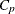statistic

EDF.

error degrees of freedom

GMSEP.

estimated MSE of prediction, assuming multivariate normality

IN.

number of regressors in the model not including the intercept

JP.

final prediction error

MSE.

mean squared error

NP.

number of parameters in the model (including the intercept)

PC.

Amemiya’s prediction criterion

RMSE.

root MSE

RSQ.

R-square

SBC.

SBC statistic

SP.

SP statistic

SSE.

error sum of squares

##### Summary of PLOT Statement Graphics Options

Table 79.8 summarizes the options available in the PLOT statement. These options are available unless the LINEPRINTER option is specified in the PROC REG statement. For complete descriptions, see the section Dictionary of PLOT Statement Options.

Option

Description

General Graphics Options

Specifies the annotate data set

Requests a reference line formodel selection criteria

Requests a reference line for themodel selection criterion

Requests plots of% confidence intervals for the mean

Specifies a description for graphics catalog member

Names the plot in the graphics catalog

Overlays plots from the same model

Requests plots of% prediction intervals for individual
responses

Requests the ridge trace for ridge regression

Axis and Legend Options

Specifies LEGEND statement to be used

Suppresses display of the legend

Specifies tick mark values for horizontal axis

Specifies tick mark values for vertical axis

Reference Line Options

Specifies reference lines perpendicular to horizontal axis

Specifies line style for HREF= lines

Specifies line style for lines displayed by default

Specifies line style for VREF= lines

Suppresses display of any default reference line

Specifies reference lines perpendicular to vertical axis

Color Options

Specifies color for axis line and tick marks

Specifies color for frame

Specifies color for HREF= lines

Specifies color for lines displayed by default

Specifies color for text

Specifies color for VREF= lines

Options for Displaying the Fitted Model Equation

Specifies font of model equation and model label

Specifies text height of model equation and model label

Specifies model label

Suppresses display of the fitted model and the label

Options for Displaying Statistics in the Plot Margin

Displays Akaike’s information criterion

Displays Sawa’s Bayesian information criterion

Displays Mallows’statistic

Displays the error degrees of freedom

Displays the estimated MSE of prediction assuming
multivariate normality

Displays the number of regressors in the model not including
the intercept

Displays the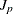statistic

Displays the mean squared error

Suppresses display of the default statistics: the number of
root mean square error

Displays the number of parameters in the model including the
intercept, if any

Displays the PC statistic

Displays the SBC statistic

Displays the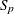statistic

Displays the error sum of squares

Specifies font of text displayed in the margin

Specifies height of text displayed in the margin

##### Dictionary of PLOT Statement Options

The following entries describe the PLOT statement options in detail. Note that these options are available unless you specify the LINEPRINTER option in the PROC REG statement.

AIC

displays Akaike’s information criterion in the plot margin.

ANNOTATE=SAS-data-set
ANNO=SAS-data-set

specifies an input data set that contains appropriate variables for annotation. This applies only to displays created with the current PLOT statement. See SAS/GRAPH: Reference for more information.

BIC

displays Sawa’s Bayesian information criterion in the plot margin.

CAXIS=color
CAXES=color
CA=color

specifies the color for the axes, frame, and tick marks.

CFRAME=color
CFR=color

specifies the color for filling the area enclosed by the axes and the frame.

CHOCKING=color

requests reference lines corresponding to the equations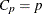and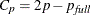, where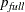is the number of parameters in the full model (excluding the intercept) and p is the number of parameters in the subset model (including the intercept). The color must be specified; theline is solid and theline is dashed. Only PLOT statements of the form PLOT CP.*NP. produce these lines.

For the purpose of parameter estimation, Hocking (1976) suggests selecting a model where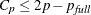. For the purpose of prediction, Hocking suggests the criterion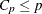. You can request the single reference linewith the CMALLOWS= option. If, for example, you specify both CHOCKING=RED and CMALLOWS=BLUE, then theline is red and theline is blue.

CHREF=color
CH=color

specifies the color for lines requested with the HREF= option.

CLINE=color
CL=color

specifies the color for lines displayed by default. See the NOLINE option for details.

CMALLOWS=color

requests areference line, where p is the number of parameters (including the intercept) in the subset model. The color must be specified; the line is solid. Only PLOT statements of the form PLOT CP.*NP. produce this line.

Mallows (1973) suggests that all subset models withsmall and near p  be considered for further study. See the CHOCKING= option for related model-selection criteria.

CONF

is a keyword used as a shorthand option to request plots that include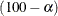% confidence intervals for the mean response. The ALPHA= option in the PROC REG or MODEL statement selects the significance level, which is 0.05 by default. The CONF option is valid for simple regression models only, and is ignored for plots where confidence intervals are inappropriate. The CONF option replaces the CONF95 option; however, the CONF95 option is still supported when the ALPHA= option is not specified. The OVERLAY option is ignored when the CONF option is specified.

CP

displays Mallows’statistic in the plot margin.

CTEXT=color
CT=color

specifies the color for text including tick mark labels, axis labels, the fitted model label and equation, the statistics displayed in the margin, and legends.

CVREF=color
CV=color

specifies the color for lines requested with the VREF= option.

DESCRIPTION=’string
DESC=’string

specifies a descriptive string, up to 40 characters, that appears in the description field of the PROC GREPLAY master menu.

EDF

displays the error degrees of freedom in the plot margin.

GMSEP

displays the estimated mean square error of prediction in the plot margin. Note that the estimate is calculated under the assumption that both independent and dependent variables have a multivariate normal distribution.

HAXIS=values
HA=values

specifies tick mark values for the horizontal axis.

HREF=values

specifies where reference lines perpendicular to the horizontal axis are to appear.

IN

displays the number of regressors in the model (not including the intercept) in the plot margin.

JP

displays thestatistic in the plot margin.

LEGEND=LEGENDn

specifies the LEGENDn statement to be used. The LEGENDn statement is a global graphics statement; see SAS/GRAPH: Reference for more information.

LHREF=linetype
LH=linetype

specifies the line style for lines requested with the HREF= option. The default linetype is 2. Note that LHREF=1 requests a solid line. See SAS/GRAPH: Reference for a table of available line types.

LLINE=linetype
LL=linetype

specifies the line style for reference lines displayed by default; see the NOLINE option for details. The default linetype is 2. Note that LLINE=1 requests a solid line.

LVREF=linetype
LV=linetype

specifies the line style for lines requested with the VREF= option. The default linetype is 2. Note that LVREF=1 requests a solid line.

MODELFONT=font

specifies the font used for displaying the fitted model label and the fitted model equation. See SAS/GRAPH: Reference for tables of software fonts.

MODELHT=height

specifies the text height for the fitted model label and the fitted model equation.

MODELLAB=’label

specifies the label to be displayed with the fitted model equation. By default, no label is displayed. If the label does not fit on one line, it is not displayed. See the section Traditional Graphics for more information.

MSE

displays the mean squared error in the plot margin.

NAME=’string

specifies a descriptive string, up to eight characters, that appears in the name field of the PROC GREPLAY master menu. The default string is REG.

NOLEGEND

suppresses the display of the legend.

NOLINE

suppresses the display of default reference lines. A default reference line at zero is displayed if residuals are plotted. If the dependent variable is plotted against the independent variable in a simple regression model, then the fitted regression line is displayed by default. Default reference lines are not displayed if the OVERLAY option is specified.

NOMODEL

suppresses the display of the fitted model equation.

NOSTAT

suppresses the display of statistics in the plot margin. By default, the number of observations, R-square, adjusted R-square, and root MSE are displayed.

NP

displays the number of regressors in the model including the intercept, if any, in the plot margin.

OVERLAY

overlays all plots specified in the PLOT statement from the same model on one set of axes. The variables for the first plot label the axes. The procedure automatically scales the axes to fit all of the variables unless the HAXIS= or VAXIS= option is used. Default reference lines are not displayed. A default legend is produced; the LEGEND= option can be used to customize the legend.

PC

displays the PC statistic in the plot margin.

PRED

is a keyword used as a shorthand option to request plots that include% prediction intervals for individual responses. The ALPHA= option in the PROC REG or MODEL statement selects the significance level, which is 0.05 by default. The PRED option is valid for simple regression models only, and is ignored for plots where prediction intervals are inappropriate. The PRED option replaces the PRED95 option; however, the PRED95 option is still supported when the ALPHA= option is not specified. The OVERLAY option is ignored when the PRED option is specified.

RIDGEPLOT

creates overlaid plots of ridge estimates against ridge values for each coefficient. The points corresponding to the estimates of each coefficient in the plot are connected by lines. For ridge estimates to be computed and plotted, the OUTEST= option must be specified in the PROC REG statement, and the RIDGE=list must be specified in either the PROC REG or MODEL statement.

SBC

displays the SBC statistic in the plot margin.

SP

displays thestatistic in the plot margin.

SSE

displays the error sum of squares in the plot margin.

STATFONT=font

specifies the font used for displaying the statistics that appear in the plot margin. See SAS/GRAPH: Reference for tables of software fonts.

STATHT=height

specifies the text height of the statistics that appear in the plot margin.

USEALL

specifies that predicted values at data points with missing dependent variable(s) be included on appropriate plots. By default, only points used in constructing the SSCP matrix appear on plots.

VAXIS=values
VA=values

specifies tick mark values for the vertical axis.

VREF=values

specifies where reference lines perpendicular to the vertical axis are to appear.

#### Line Printer Plots

Line printer plots are requested with the LINEPRINTER option in the PROC REG statement. Points in line printer plots can be marked with symbols, which can be specified as a single character enclosed in quotes or the name of any variable in the input data set.

If a character variable is used for the symbol, the first (leftmost) nonblank character in the formatted value of the variable is used as the plotting symbol. If a character in quotes is specified, that character becomes the plotting symbol. If a character is used as the plotting symbol, and if there are different plotting symbols needed at the same point, the symbol ’?’ is used at that point.

If an unformatted numeric variable is used for the symbol, the symbols ’1’, ’2’, …, ’9’ are used for variable values 1, 2, …, 9. For noninteger values, only the integer portion is used as the plotting symbol. For values of 10 or greater, the symbol ’*’ is used. For negative values, a ’?’ is used. If a numeric variable is used, and if there is more than one plotting symbol needed at the same point, the sum of the variable values is used at that point. If the sum exceeds 9, the symbol ’*’ is used.

If a symbol is not specified, the number of replicates at the point is displayed. The symbol ’*’ is used if there are 10 or more replicates.

If the LINEPRINTER option is used, you can specify the following options in the PLOT statement after a slash (/):

CLEAR

clears any collected scatter plots before plotting begins but does not turn off the COLLECT option. Use this option when you want to begin a new collection with the plots in the current PLOT statement. For more information about collecting plots, see the COLLECT and NOCOLLECT options in this section.

COLLECT

specifies that plots begin to be collected from one PLOT statement to the next and that subsequent plots show an overlay of all collected plots. This option enables you to overlay plots before and after changes to the model or to the data used to fit the model. Plots collected before changes are unaffected by the changes and can be overlaid on later plots. You can request more than one plot with this option, and you do not need to request the same number of plots in subsequent PLOT statements. If you specify an unequal number of plots, plots in corresponding positions are overlaid. For example, the statements

plot residual.*predicted. y*x / collect;
run;


produce two plots. If these statements are then followed by

plot residual.*x;
run;


two plots are again produced. The first plot shows residual against X values overlaid on residual against predicted values. The second plot is the same as that produced by the first PLOT statement.

Axes are scaled for the first plot or plots collected. The axes are not rescaled as more plots are collected.

Once specified, the COLLECT option remains in effect until the NOCOLLECT option is specified.

HPLOTS=number

sets the number of scatter plots that can be displayed across the page. The procedure begins with one plot per page. The value of the HPLOTS= option remains in effect until you change it in a later PLOT statement. See the VPLOTS= option for an example.

NOCOLLECT

specifies that the collection of scatter plots ends after adding the plots in the current PLOT statement. PROC REG starts with the NOCOLLECT option in effect. After you specify the NOCOLLECT option, any following PLOT statement produces a new plot that contains only the plots requested by that PLOT statement.

OVERLAY

enables requested scatter plots to be superimposed. The axes are scaled so that points on all plots are shown. If the HPLOTS= or VPLOTS= option is set to more than one, the overlaid plot occupies the first position on the page. The OVERLAY option is similar to the COLLECT option in that both options produce superimposed plots. However, OVERLAY superimposes only the plots in the associated PLOT statement; COLLECT superimposes plots across PLOT statements. The OVERLAY option can be used when the COLLECT option is in effect.

SYMBOL=’character

changes the default plotting symbol used for all scatter plots produced in the current and in subsequent PLOT statements. Both SYMBOL=” and SYMBOL=’ ’ are allowed.

If the SYMBOL= option has not been specified, the default symbol is ’1’ for positions with one observation, ’2’ for positions with two observations, and so on. For positions with more than 9 observations, ’*’ is used. The SYMBOL= option (or a plotting symbol) is needed to avoid any confusion caused by this default convention. Specifying a particular symbol is especially important when either the OVERLAY or COLLECT option is being used.

If you specify the SYMBOL= option and use a number for character, that number is used for all points in the plot. For example, the statement

plot y*x / symbol='1';


produces a plot with the symbol ’1’ used for all points.

If you specify a plotting symbol and the SYMBOL= option, the plotting symbol overrides the SYMBOL= option. For example, in the statements

plot y*x y*v='.' / symbol='*';


the symbol used for the plot of Y against X is ’*’, and a ’.’ is used for the plot of Y against V.

If a paint symbol is defined with a PAINT statement, the paint symbol takes precedence over both the SYMBOL= option and the default plotting symbol for the PLOT statement.

VPLOTS=number

sets the number of scatter plots that can be displayed down the page. The procedure begins with one plot per page. The value of the VPLOTS= option remains in effect until you change it in a later PLOT statement.

For example, to specify a total of six plots per page, with two rows of three plots, use the HPLOTS= and VPLOTS= options as follows:

plot y1*x1 y1*x2 y1*x3 y2*x1 y2*x2 y2*x3 /
hplots=3 vplots=2;
run;